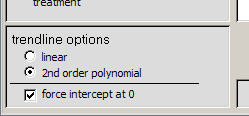﻿ Making Graphs > Standard Graphs > Predictability
 Navigation:  Making Graphs > Standard Graphs > PredictabilityAttempted vs. Achieved SEQ Scatter GraphLooking at mean values alone will not tell you the true distribution of your results. Outliers to both sides may sum up perfectly at zero, but what counts for refractive surgery patients is the predictability of ending up at a desired target. A scatter plot like the above (a bad example of highly scattered outcomes) may be useful to get an idea about the actual risk of ending up over or undercorrected. The more eyes are shown in between the dashed ±0.5 and ± 1.0 D  lines, the better. The graph title contains the namer of eyes and follow up interval. The top right text box contains the average deviation from target and the ranges.

When this graph is opened and at least one eye is outside a +-2 D range, a warning window will appear, followed by a sorted list of postOP results. You can open the data entry form of such outliers, by double click on the treatment ID number.The filter window for scatter plot graphs, shows 'trendline options. Depending on the type and amount of data you may switch between linear or second order polynomial regression, forcing intercept at zero or not. By default it is set to linear and force intercept at 0.Most scatter graphs will display a 'regression formula' (y= *x² + *x + t) and a 'coefficient of determination' (R²). The numbers before the x² describe the non linearity ('curve') of the trendline. The slope of the data is given before the 'x' and any offset as t. The coefficient of determination 'R²' describes the quality of the 'fit' of the regression analysis. A value between 0.9 and 1.0 represents a good fit, otherwise your data is significantly scattered  and not well represented by the regression formula.